Private GMAT Tutoring in NYC & Online

# GMAT Question of the Day - Problem Solving - Rate

A driver completed the first half of a trip at an average speed of 30 miles per hour. What speed must the driver average for the second half of the trip in order to average a speed of 36 miles per hour for the entire journey (assuming that the driver did not make any stops during the trip)?

A. 42

B. 45

C. 48

D. 50

E. 52

# GMAT Question of the Day Solution

This is a classic GMAT rate question: average speed for a two part journey. Let's remember that with average rate you can't simply take the average of the rates because doing so assumes that you spent an equal amount of time driving at each rate. Usually that's not the case so you need to add a "weight" to each of the rates before combining them. The "weight" is how much time you spent driving at that rate.

The general strategy for GMAT weighted average/rate questions is to make a T for each part of the journey. In this case you would have the two pieces and the total. The time boxes for each of the pieces sum to the total time. The distance boxes sum to the total distance. Divide the total distance by the total time to solve for the "total" rate.

In this question you are given the total rate and need to work backwards in order to solve the rate for the second half of the journey. The procedure is the same. Work with the time and distance in order to calculate the rate. We are not given a number for the total distance but we can certainly pick a number so that the question comes more into focus. Pick a number that works nicely with the given information. In this case a multiple of 180 will work well with 30 and 36. Here's an Official GMAT question on which we also use the T method: A boat traveled upstream a distance of 90 miles at an average speed of (v-3) miles per hour and then traveled the same distance downstream at an average speed of (v+3) miles per hour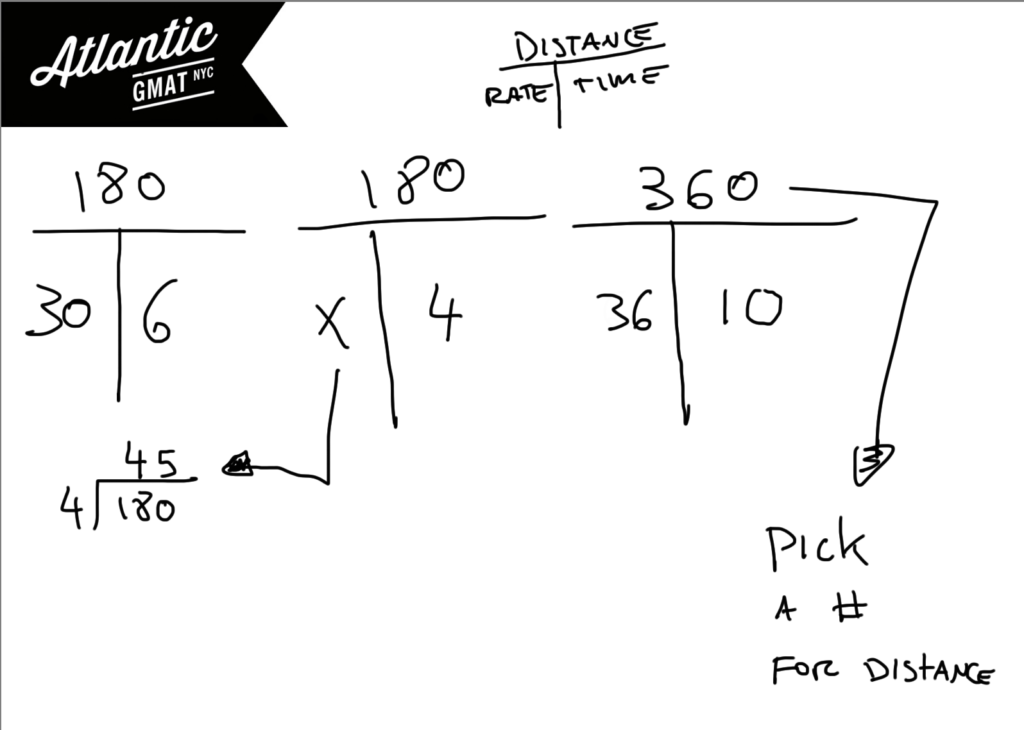Here are more GMAT average rate practice questions:

Distance, Work and Rate - Average Rate 1

Distance, Work and Rate - Average Rate 2

For more GMAT distance, work, and rate practice visit GMAT Question of the Day.

# GMAT Question of the Day - Problem Solving - Work

Tank A contains X gallons of water while Tank B contains Y gallons. Tank A has water removed at a rate of K gallons per hour and Tank B has water added at a rate of M gallons per hour. Assuming that both tanks still contain some water, in terms of X, Y, K, and M how many gallons of water will both tanks have 2 minutes from now?

A.  (x - y - m + k)/60

B. (30(x-y) - m + k)/60

C. (30(x-y) + m - k)/60

D. (30(x-y) + m - k)/30

E. (30(x-y) - m + k)/30

## GMAT Question of the Day Solution

For GMAT work and rate questions it helps to organize the information in T's. This way you have a place to store the numbers in a way that identifies what the numbers represent. The T's also help you make inferences. Notice that in this question there is a unit conversion from minutes to hours. For GMAT work and rate questions it's a great idea to double check the units before committing to an answer.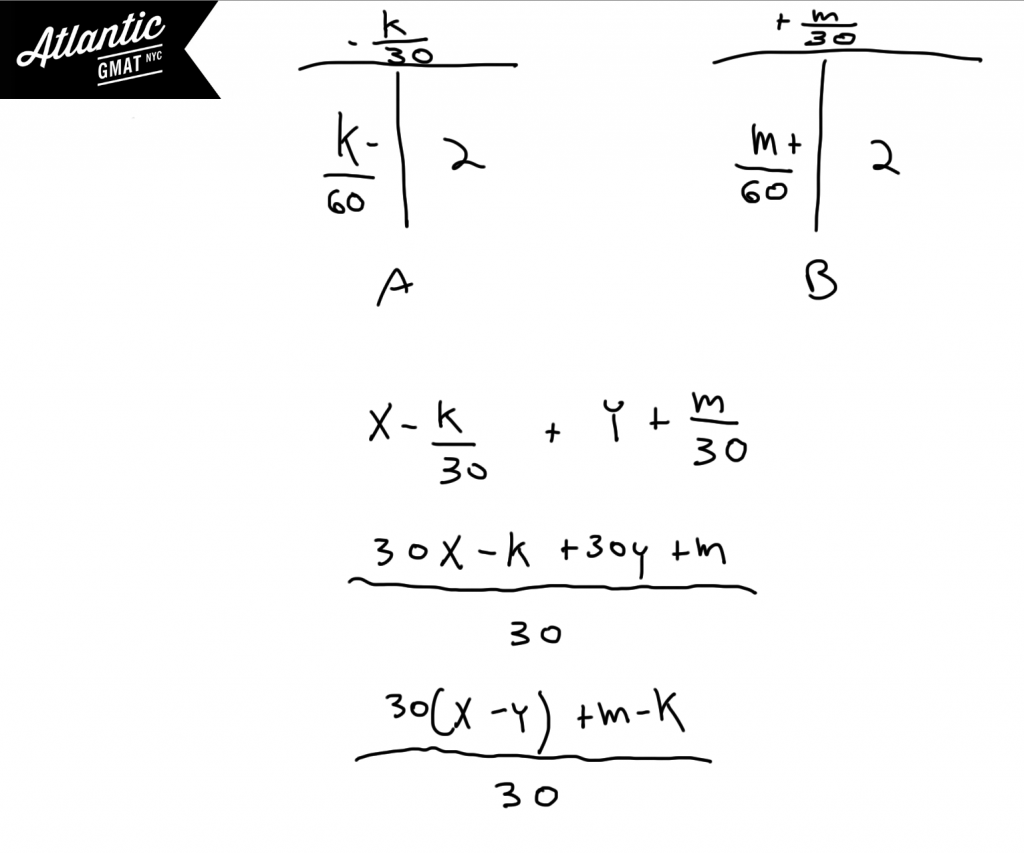# GMAT Question of the Day - Problem Solving - Work/Rate

Worker A and Worker B working together can paint 31 walls per day. If Worker B increases his rate by 1/3 the two of them could paint 34 walls per day. How many walls can Worker A paint in a day?

A. 9

B. 15

C. 19

D. 20

E. 22

## GMAT Question of the Day Solution

This work question may seem a slight bit out of the ordinary but you can tackle it the same way as you would tackle any other work/rate question. Make T's to organize the information and then work your way towards what you are solving for. In this case we are looking for the rate of Worker A. We are given some information about the two people working together and some information about Worker B. Most likely we'll be subtracting the rate of Worker B from the combined rate to get the Rate of Worker A. In this question you're not directly given the rate of Worker B but you're really only one step removed from figuring that out.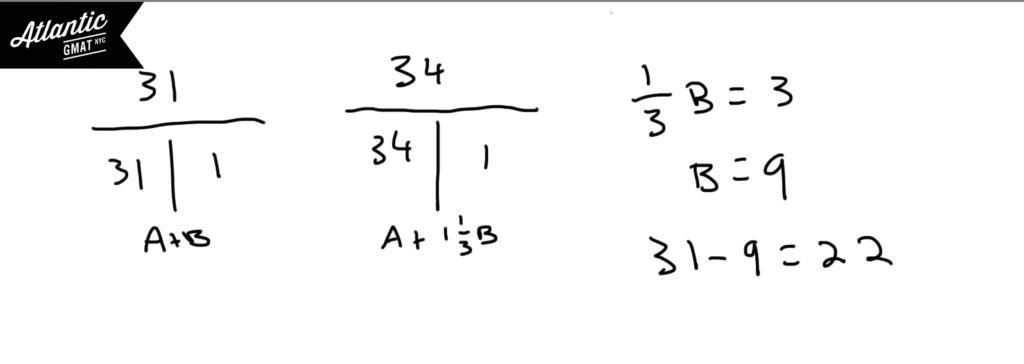If you are still a little confused about how we figured out that the rate of Worker B is equal to 9 you might consider setting up some parallel equations. Then you can subtract out the rate of Worker A and solve for the rate of Worker B. Here's an Official GMAT cooperative distance, work, and rate question to practice on: Pumps A, B, and C operate at their respective constant rates...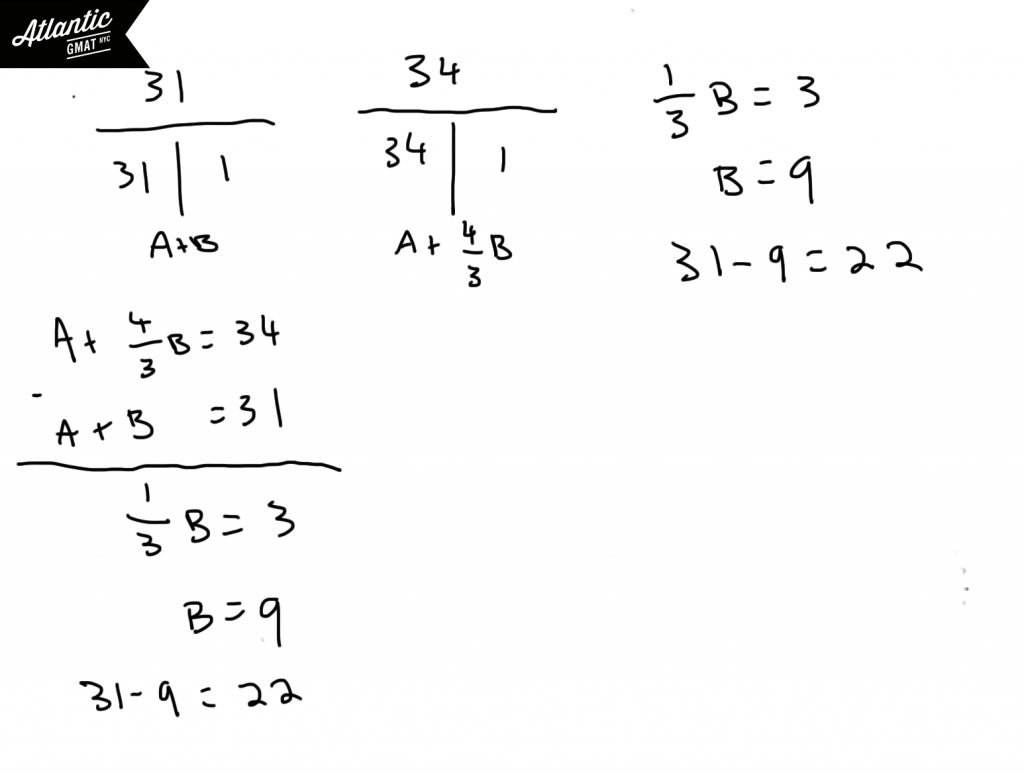Here are another bunch of cooperative work and rate questions to practice on:

Work and Rate 1

Work and Rate 2

Work and Rate 3

Work and Rate 4

Work and Rate 5

For more distance, work, and rate examples you can also check out our GMAT Question of the Day page

# GMAT Question of the Day - Problem Solving - Work and Rate

Paul and Janice are headed directly towards each other with Paul traveling at a rate 5/7ths that of Janice. If Janice can cover the current distance between them in 120 hours and if they maintain constant speed and direction then in how many hours will they meet?

A. 70

B. 72

C. 74

D. 75

E. 77

Here's an Official GMAT distance, work, and rate question and explanation to practice on: Pumps A, B, and C operate at their respective constant rates...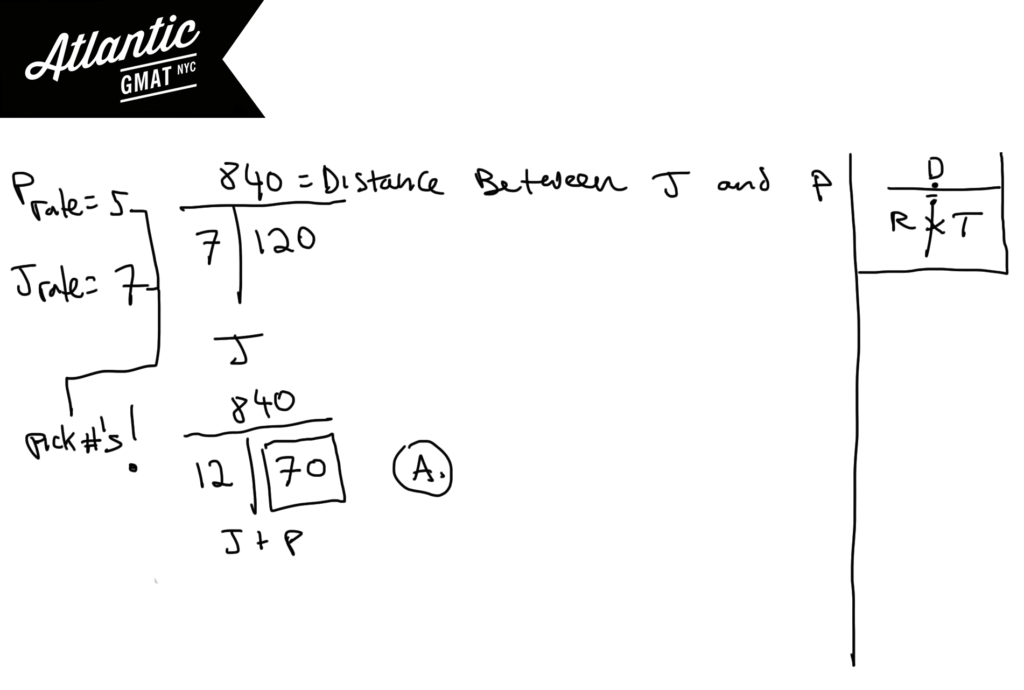Here are another bunch of cooperative work and rate questions to practice on:

Work and Rate 1

Work and Rate 2

Work and Rate 3

Work and Rate 4

Work and Rate 5

For more distance, work, and rate examples you can also check out our GMAT Question of the Day page

# GMAT Question of the Day - Problem Solving - Rate

Peter and Jane were both driving at 60mph down a certain highway heading south. At 3pm Peter crossed the border into Mexico and decreased his speed by 10mph. If Jane crossed the border into Mexico at 3:45pm, how many hours after crossing into Mexico will it take her to catch up with Peter?

A. 2.5

B. 3.5

C. 3.75

D. 4.5

E. 5

## GMAT Question of the Day Solution:

A rate T will be mighty helpful on this question. Take your time on GMAT word problems. Often they are not terribly complicated but it is easy to misinterpret the information throwing the whole approach into chaos.

First, read and understand the information. Then define what you are solving for. Lastly, use the details to fill in the blanks. This question is about relative rate - the rate at which Jane is catching up with Peter. In a chase scenario (one person stalking another) you subtract the rates. If people are headed towards each other (two cars crashing head on) you add the rates. This is the first scenario. Jane is trying to catch up with Peter. Here's an Official GMAT cooperative distance, work, and rate question to practice on: Pumps A, B, and C operate at their respective constant rates...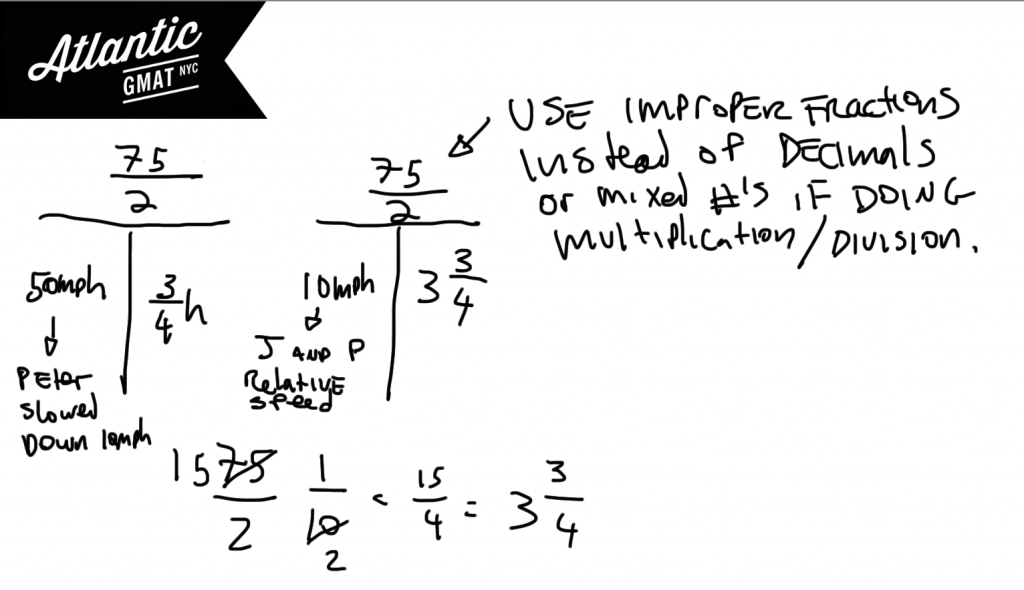Here are another bunch of cooperative work and rate questions to practice on:

Work and Rate 1

Work and Rate 2

Work and Rate 3

Work and Rate 4

Work and Rate 5

For more distance, work, and rate examples you can also check out our GMAT Question of the Day page

# CONTACT

Atlantic GMAT

405 East 51st St.

NY, NY 10022

(347) 669-3545

info@AtlanticGMAT.com